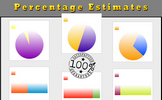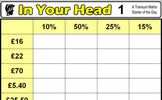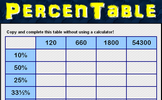# Percentages

### Term 2 starting in week 3 :: Estimated time: 2 weeks

• Understand percentages (review)
• Fractions to percentages
• Equivalent FDP
• Order FDP
• Percentage of an amount (1)
• Percentage of an amount (2)
• Percentages - missing values

This page should remember your ticks from one visit to the next for a period of time. It does this by using Local Storage so the information is saved only on the computer you are working on right now.

## Lesson Starters

Here are some suggestions for whole-class, projectable resources which can be used at the beginnings of each lesson in this block.

### 1st Lesson#### Estimating Percentages

Estimate the percentages of full circles and rectangles the sectors represent.

### 2nd Lesson#### Fractions Decimals Percentages

Convert fractions to decimals, decimals to percentages and percentages to fractions.

### 3rd LessonHere are the simple percentage calculations everyone should be able to do in their heads.

### 4th Lesson#### Quick Percentages

Simple percentage questions appear on screen then fade every 8 seconds. This Starter is customisable.

### 5th Lesson#### PercenTable

Complete the table by calculating common percentages without using a calculator.

### 6th Lesson#### Refreshing Revision

It is called Refreshing Revision because every time you refresh the page you get different revision questions.

Some of the Starters above are to reinforce concepts learnt, others are to introduce new ideas while others are on unrelated topics designed for retrieval practice or and opportunity to develop problem-solving skills.

White Rose ResourcesEnd of block assessments provide a quick progress check at the end of each block of learning to make sure students have understood the content covered. This Scheme of Learning was produced by White Rose Maths and is used here with permission granted on 30th June 2021.For All: